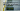# Maximum Length of Subarray With Positive Product - Leet Code Solution

August 31, 2020## Problem Statement

Maximum Length of Subarray With Positive Product.

Given an array of integers nums, find the maximum length of a subarray where the product of all its elements is positive.

A subarray of an array is a consecutive sequence of zero or more values taken out of that array.

Return the maximum length of a subarray with positive product.

Example

``````Input: nums = [1,-2,-3,4]
Output: 4

Input: nums = [0,1,-2,-3,-4]
Output: 3``````

Note: You can include value `0` in your range as this will make product zero.

## Solution

Note: We don’t have to include value-0 in our result. This adds to additional complexity to problem.

Lets think of sliding window kind of solution where we keep track of some indexes and calculate the result.

Few things to keep in mind:

• You can not leave a negative number as it is.
As multiplication of two negative numbers is positive
• You don’t need to actually multiply numbers. The question is about maximum subarray length which multiplies to a positive result.
• You just need to keep a track of how many numbers multiplies to a positive result.
• If your negative counts becomes odd, you would want to remove a negative number from your range.

### Lets talk about some helper variables

Lets talk about some of the variables we will need in this problem.

Negative Count
You need a variable to keep the count the negatives till your iterator index.

Index of Zero
You will need an index of when you last found a `zero`.

Index of first negative number
This will be required in case you encountered negative count to be odd. You would want to calculate length of array excluding first negative number.

### Code

Alright, lets look at the code.

``````public int getMaxLen(int[] nums) {
int max = 0;

int firstNegativeIndex = -1;
int negativeCount = 0;

int zeroIndex = -1;

for (int i=0; i<nums.length; i++) {
//{-1,-2,-3,0,1};
if (nums[i] < 0) {
negativeCount ++;
//update firstNegativeIndex for first time
if (firstNegativeIndex == -1) {
firstNegativeIndex = i;
}
else {
//if its a negative number and count becomes even
if (negativeCount % 2 == 0) {
max = Math.max(max, i-zeroIndex);
}
}
}
else if (nums[i] == 0) {
//reset everything
firstNegativeIndex = -1;
negativeCount = 0;
zeroIndex = i;
}
else {
if (negativeCount % 2 == 0) {
max = Math.max(max, i-zeroIndex);
}
else {
max = Math.max(max, i-firstNegativeIndex);
}
}
}

return max;
}``````

### Complexity

Its `O(n)`